Multiple Choice
Identify the choice that best completes the statement or answers the question.

1.

Computation – Fractions – RIT 211 – 220

Write 6/12 in simplest form.
 a. 1/2 d. 1/6 b. 12/24 e. 1/6 c. 2/4

2.

2/10 + 4/10 =
 a. 3/10 d. 6/20 b. 3/5 e. 6/10 c. 2/5

3.

1 7/8 + 3 3/4 =
 a. 4 10/12 d. 5 3/4 b. 4 7/8 e. 45/8 c. 5 5/8

4.

Multiply and simplify.
5 x 1/3 =
 a. 15/9 d. 1 2/3 b. 1 6/9 e. 14/3 c. 16/3

5.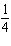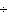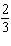=
 a. 3/7 d. 1 b. 11/12 e. 3/8 c. 2/12

6.

3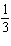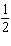=
 a. 6 2/3 d. 20/3 b. 3 5/6 e. 6 1/2 c. 2 5/6

7.

Order each fraction from least to greatest.
1/2, 1/3, 5/6, 2/3, 1/6
 a. 5/6, 1/6, 2/3, 1/3, 1/2 d. 1/6, 1/3, 1/2, 2/3, 5/6 b. 5/6, 2/3, 1/2, 1/3, 1/ e. 1/2, 1/3, 5/6, 2/3, 1/6 c. 5/6, 2/3, 1/6, 1/3, 1/

8.

Computation – Integers – RIT 211 – 220

Which statement is true?
a = 12
b = 21
 a. b < a d. b > a b. b < a e. 9 c. 34

9.

–6 + (-7) =
 a. +13 d. 1 b. –1 e. –13 c. 42

10.

5 + (-15) =
 a. 10 d. –10 b. –20 e. –75 c. 20

11.

6 + (-2) + 3 + (-5) =
 a. 2 d. 6 b. 16 e. -2 c. –16

12.

Multiply these two integers.
(-8) (6) =
 a. 14 d. –48 b. –14 e. –2 c. 48

13.

- 246 = ___
 a. 4 d. –18 b. –4 e. -30 c. 18

14.

Computation – Whole Numbers – RIT 211 – 220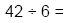a. 6 x 6 = 42 d. 7 < 42 b. 42 > 6 e. 6 x 7 = 42 c. 7 ¸ 6 = 42

15.

3,0476 - ___
 a. 3,647 d. 507 R5 b. 500 R7 e. 57 R5 c. 18,282

16.

When you multiply any number by 10 you add a ______ to that number.
 a. 10 d. 00 b. 0 e. decimal c. 1

17.

8,53276 = ____
 a. 8,456 d. 101 R20 b. 1,120 e. 112 R20 c. 8,608